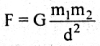Physics

# What are the fundamental forces?

The force whose actions-reactions are seen in nature is called fundamental forces. In other words, those forces which are fundamental and do not originate from other forces, but all forces can be expressed in terms of those forces are called fundamental forces. All the forces in nature are classified into four- classes: viz

1. Gravitational force
2. Weak nuclear force
3. Electromagnetic force
4. Strong nuclear force

Description of the forces:

1. Gravitational force: In this universe, every particle of matters attracts every other particle with a force. This force is called Gravitational force. This force effects due to the particle graviton of the two bodies. They are interchanged with each other. According to the law of gravitation,  F ∞ m1 m2  &  F ∞ 1/d2

(where m1 & m2 are masses and d is the distance)where G = Gravitational constant.

It is a weak force relative to the other three forces.

2. Weak nuclear force: The weak nuclear force is the short-range force. It is effective inside the particle Due to this force, the radioactive B-decay has occurred. It has no range.

3. Electromagnetic force: This type of force is also called coulomb’s force. This force is developed due to the attraction-repulsion in static electric charges. This force is effective due to the exchange of photon (photon has no mass & charge). This force is stronger than the above two forces.

4. Strong nuclear force: The force is seen between the two nucleons in the nuclear. This force is the strongest among all other forces known. This force does not effective outside the nuclear of the atom.

The intensities of the fundamental forces:

The four forces are described in the above, among them gravitational force is the weakest to all of them. Taking its magnitude (intensity or, strength) 1 (one), other’s intensities or, strength’s are given below:

Gravitational force = 1
Weak nuclear force = l030
Electromagnetic force = l040
Strong nuclear force = 1039

Again, taking the intensity of strong nuclear force as 1 (one), the other intensities are as follows:

Strong nuclear force = 1
Electromagnetic force = 10-2
Weak nuclear force = 10-13
Gravitational force = 10-39

The fundamental forces act or effective in the very short range.